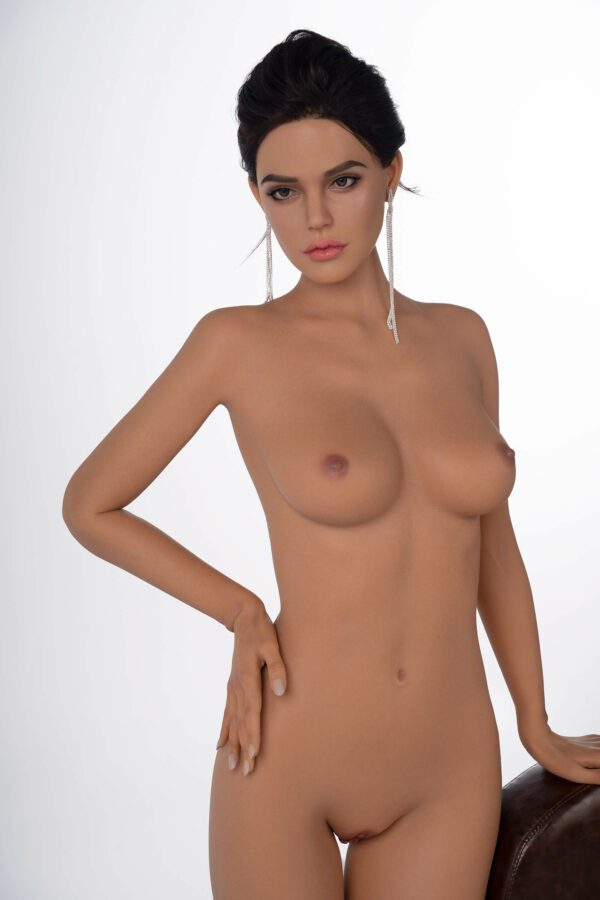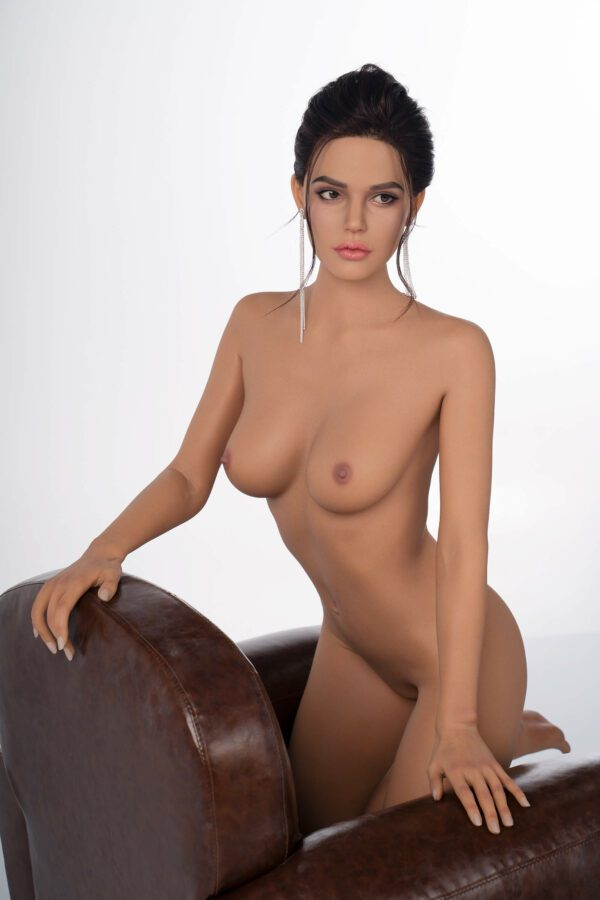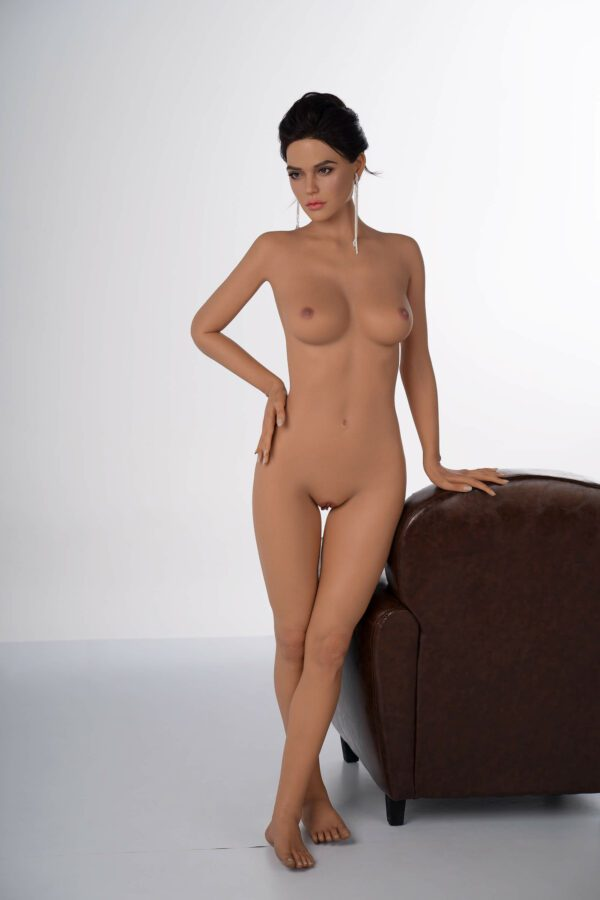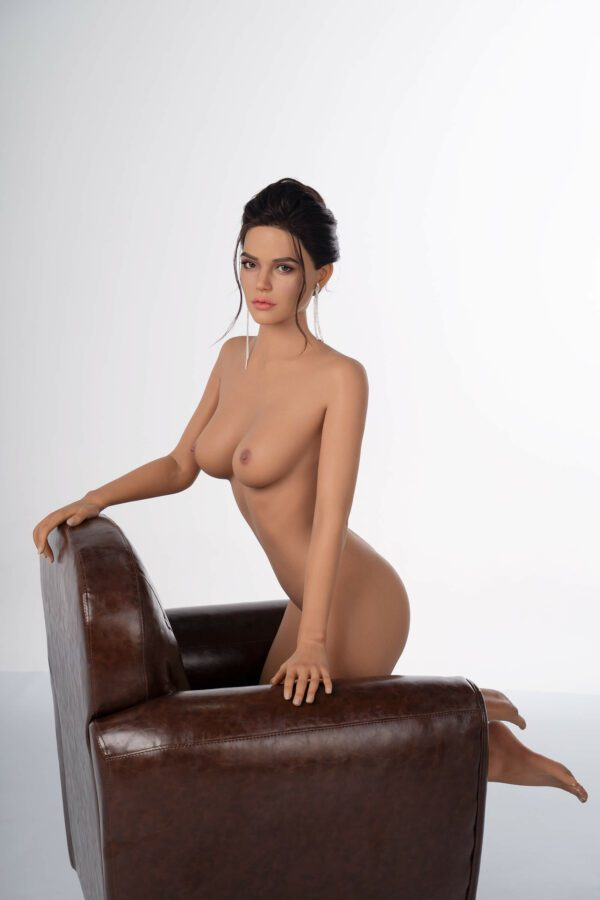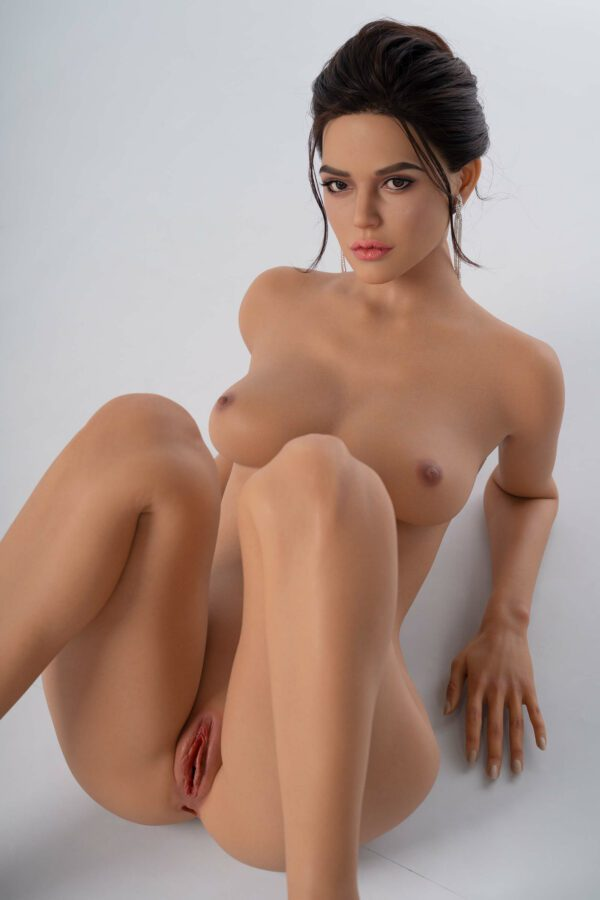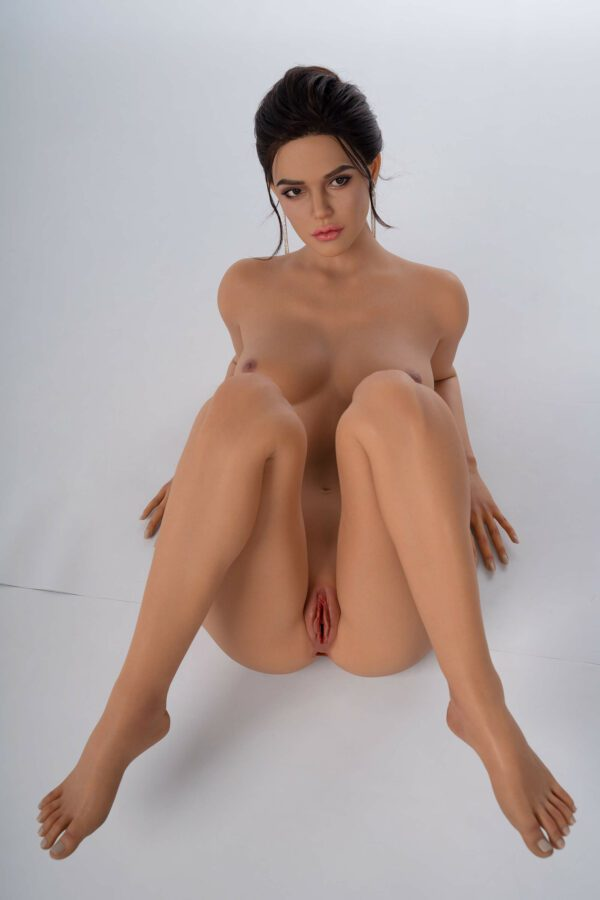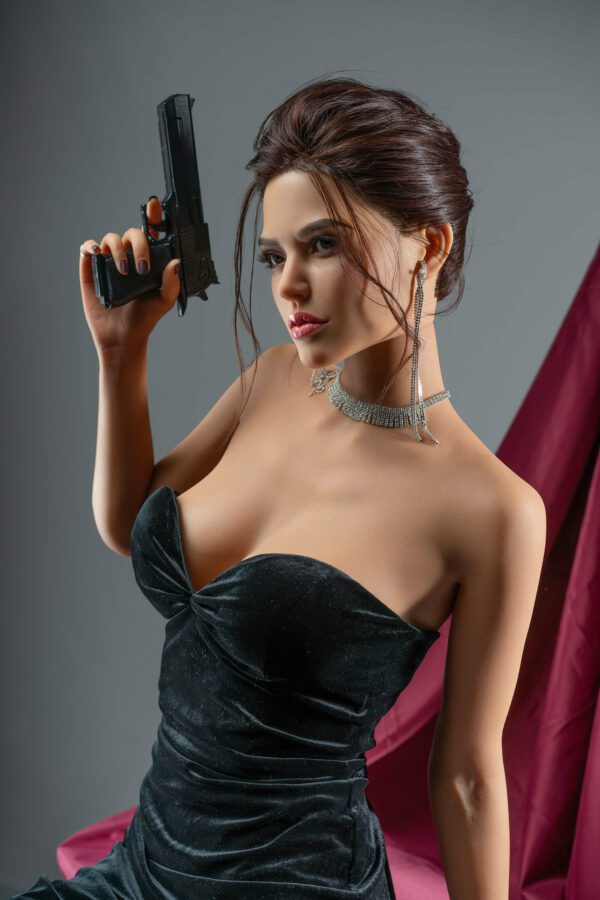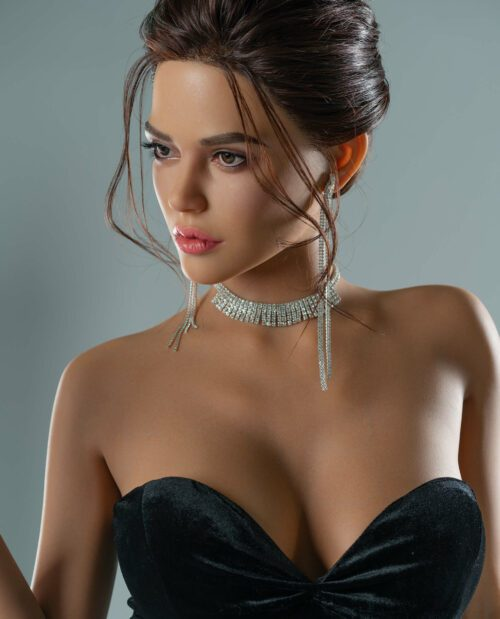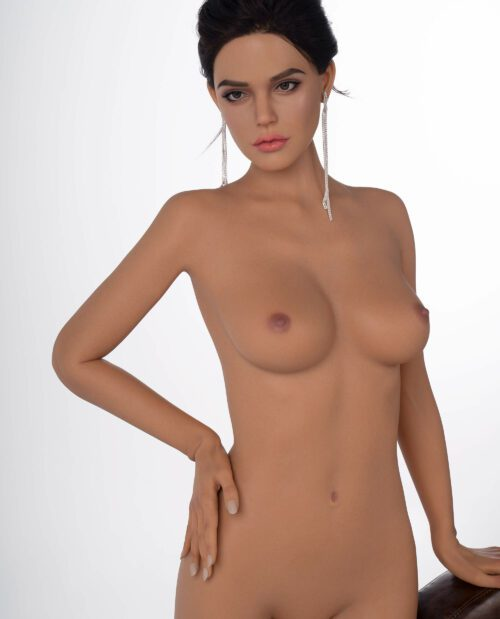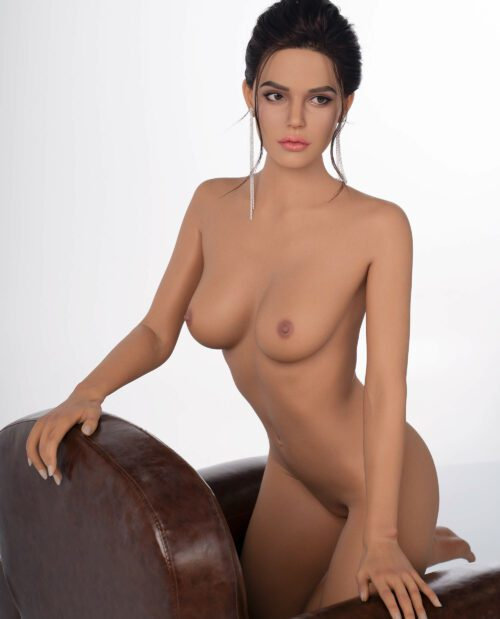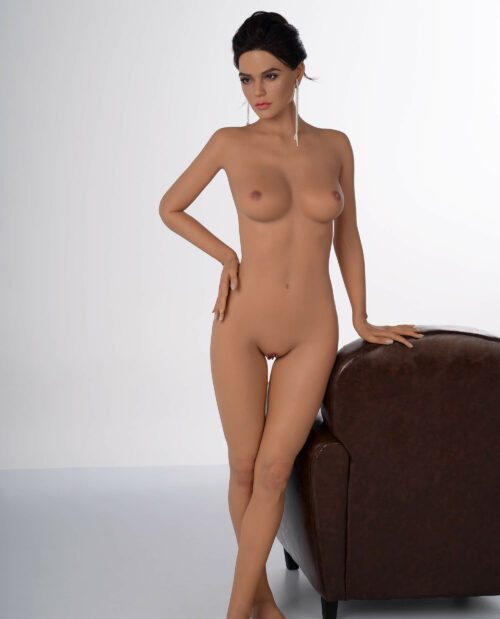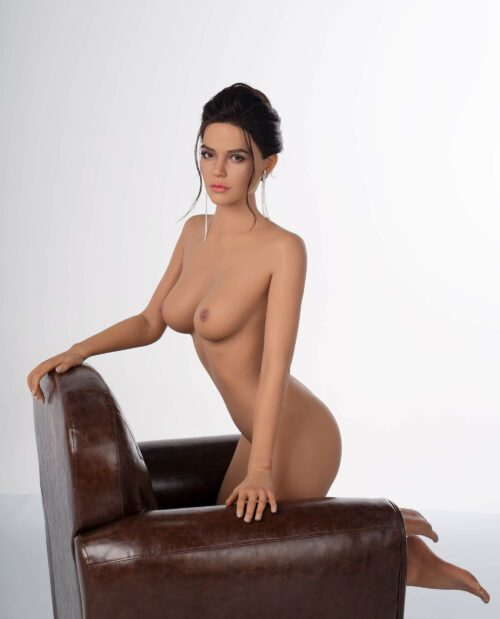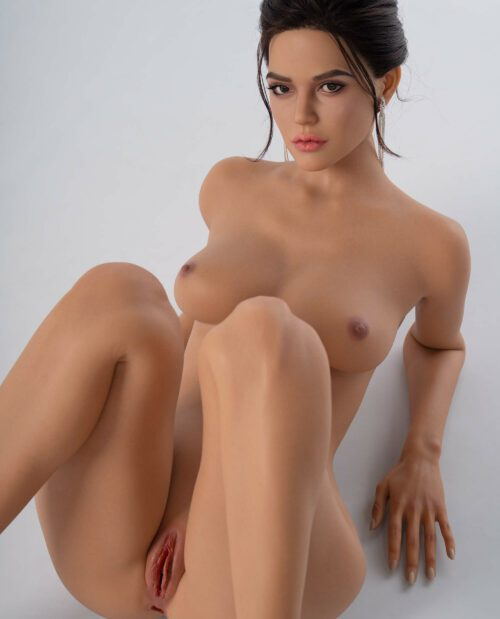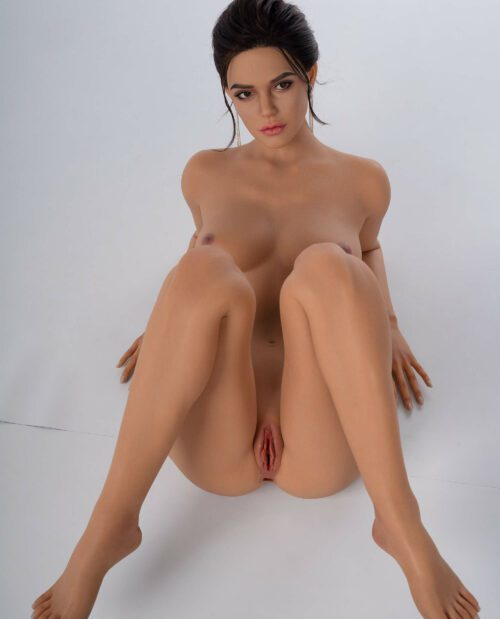# Emilia – 5’7″ | 170cm Realistic Sex doll

Zelex Doll
\$2,800

XXX

• \$
• \$
• \$
• \$
• \$
• \$
• \$
• \$
• \$
• \$
• \$
• \$
• \$
• \$
• \$
• \$
• \$
• \$
• \$
• \$
• \$
• \$

• \$
• \$
• \$
• \$
• \$
• \$
• \$

• \$

• \$
• \$
• \$
• \$
• \$
• \$
• \$
• \$
• \$
• \$
• \$
• \$
• \$
• \$
• \$
• \$
• \$
• \$
• \$

• \$
• \$
• \$
• \$
• \$
• \$
• \$
• \$
• \$
• \$
• \$
• \$
• \$
• \$
• \$
• \$
• \$
• \$

• \$
• \$
• \$
• \$
• \$
• \$
• \$
• 170 \$

• \$
• \$

• \$
• \$
• \$
• \$

• \$
• \$
• \$
• \$
• \$
• \$
• \$

• \$

• \$

• \$
• \$
• \$
• \$
• \$
• \$
• \$
• \$
• \$
• \$
• \$
• \$
• \$
• \$

• \$
• 50 \$

• \$
• \$
• \$

• \$
• \$
• \$
• \$
• \$
• \$

• \$
• \$

• \$
• \$
• \$
• \$
• \$
• \$
• \$
• \$
• \$
• \$
• \$
• \$
• \$
• \$

• \$
• \$
• \$
• \$
• \$
• \$
• \$
• 250 \$

• \$
• 80 \$

## Doll Measurements

Height: 170 cm | 5ft 7in
Cup size: C cup
Full Bust: 87.5 cm | 34.4 in
Under Bust: 71.5 cm | 28 in
Waist: 64.5 cm | 25.4 in
Hips: 94 cm | 37 in
Foot Length: 23cm | 9.1 in
Shoulder Width: 40 cm | 15.7 in
Weight: 43.2 kg | 95.2 lbs.
Vaginal Depth: 17cm | 6.7 in
Anal Depth: 16 cm | 6.3 in
Oral Depth: N/A
Package Size: 156*46*31 cm